Euler's Theory of Columns

# Euler's Theory of Columns Notes | Study Strength of Materials (SOM) - Mechanical Engineering

## Document Description: Euler's Theory of Columns for Mechanical Engineering 2022 is part of Strength of Materials (SOM) preparation. The notes and questions for Euler's Theory of Columns have been prepared according to the Mechanical Engineering exam syllabus. Information about Euler's Theory of Columns covers topics like and Euler's Theory of Columns Example, for Mechanical Engineering 2022 Exam. Find important definitions, questions, notes, meanings, examples, exercises and tests below for Euler's Theory of Columns.

Introduction of Euler's Theory of Columns in English is available as part of our Strength of Materials (SOM) for Mechanical Engineering & Euler's Theory of Columns in Hindi for Strength of Materials (SOM) course. Download more important topics related with notes, lectures and mock test series for Mechanical Engineering Exam by signing up for free. Mechanical Engineering: Euler's Theory of Columns Notes | Study Strength of Materials (SOM) - Mechanical Engineering
 1 Crore+ students have signed up on EduRev. Have you?

Columns and Struts

• A structural member subjected to an axial compressive force is called strut. As per definition strut may be horizontal, inclined or even vertical.
• The vertical strut is called a column.
Euler’s Column Theory

Assumptions of Euler's theory:
Euler's theory is based on the following assumptions:
(i) Axis of the column is perfectly straight when unloaded.
(ii) The line of thrust coincides exactly with the unstrained axis of the column.
(iii) Flexural rigidity El is uniform.
(iv) Material is isotropic and homogeneous.

Limitation of Euler’s Formula

• There is always crookedness in the column and the load may not be exactly axial.
• This formula does not take into account the axial stress and the buckling load is given by this formula may be much more than the actual buckling load.
Euler’s Buckling (or crippling load)

The maximum load at which the column tends to have lateral displacement or tends to buckle is known as buckling or crippling load. Load columns can be analysed with the Euler’s column formulas can be given as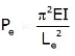where, E = Modulus of elasticity, L= Effective Length of column, and I = Moment of inertia of column section.

(i) For both end hinged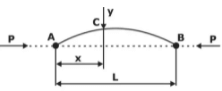in case of Column hinged at both end Le = L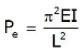(ii) For one end fixed and other free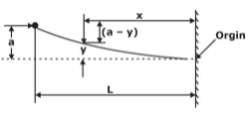in case of column one end fixed and other free: Le = 2L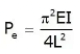(iii) For both end fixed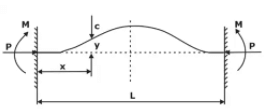in case of Column with both end Fixed Le = L / 2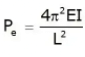(iv) For one end fixed and other hinged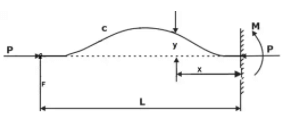in case of Column with one end fixed and other hinged Le = L / √2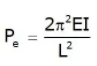(v) Effective Length for different End conditions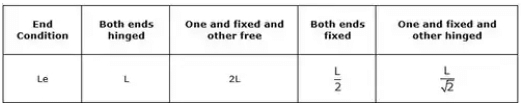Slenderness Ratio (S)

The slenderness ratio of a compression member is defined as the ratio of its effective length to least radius of gyration.

slenderness (s) = L/ k = Effective length of member / Least radius of gyration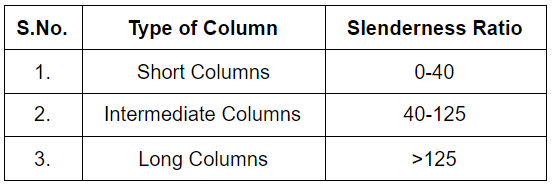Modes of failure of Columns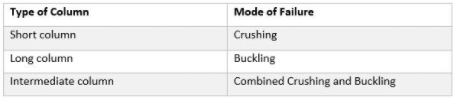Rankine’s Formula

Rankine proposed an empirical formula for columns which coven all Lasts ranging from very short to very long struts. He proposed the relation
1 / P= 1 / PC + 1 / PE

Pc = σC. A = ultimate load for a strut
Eulerian crippling load for the standard case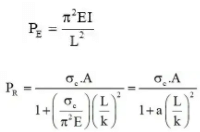Where a = Rankine's constant =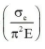The document Euler's Theory of Columns Notes | Study Strength of Materials (SOM) - Mechanical Engineering is a part of the Mechanical Engineering Course Strength of Materials (SOM).
All you need of Mechanical Engineering at this link: Mechanical Engineering

## Strength of Materials (SOM)

35 videos|34 docs|48 tests
 Use Code STAYHOME200 and get INR 200 additional OFF

## Strength of Materials (SOM)

35 videos|34 docs|48 tests

Track your progress, build streaks, highlight & save important lessons and more!

,

,

,

,

,

,

,

,

,

,

,

,

,

,

,

,

,

,

,

,

,

;